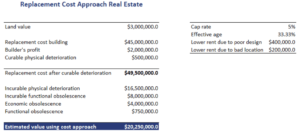# Replacement Cost Approach Real Estate

What does the cost approach mean on an appraisal? The replacement cost approach is a method to estimate the value of real estate when the property is relatively new. It is also used when valuing properties that are unusual or properties for which too few comparable transactions are available. In all of these cases, we can assume that an investor would not be willing to pay more for the property than the cost to build it plus the value of the land.

On this page, we discuss the replacement cost approach and implement a simple example of the replacement cost approach. An Excel spreadsheet that implements a simple cost approach calculator  is available at the bottom of the page.

## Replacement cost approach definition

What is the cost approach in real estate? The cost approach consists of three steps. First, we determine the value of the land. Next, we calculate the replacement cost of the building. Finally, we adjust the replacement cost for depreciation (that is, physical deterioration) and obsolescence. There are three types of obsolescence we have to consider:

• Functional obsolescence: losses resulting from defects in design
• Locational obsolescence: location is no longer optimal
• Economic obsolescence: new construction is not feasible under current economic conditions

Finally, we should also consider curable physical deterioration. These are the costs that will increase the value of the building more than they cost. These costs should be subtracted from the building cost.

The best way to understand the replacement cost approach is by going through a detailed example. This approach can easily be implemented in Excel, which we turn to now.

## Replacement Cost Approach Example

Let’s consider a simple example of the replacement cost approach to value an appraisal. The following table illustrates the method. In particular, we value a property for which we have the following data reported in the table. Using the three steps described earlier, we can easily calculate the estimated value of the property.The spreadsheet used to do the calculations is available for download below. The hard part in applying the cost approach is to make sure to subtract the curable depreciation from the replacement cost. This has to be done before applying the incurable physical deterioration.

## Summary

We discussed the real estate appraisal cost approach using a simple example. The approach consists of three steps which can easily be applied. The approach is especially easy to apply when the property is relatively new. In that case, it is easy to estimate the construction costs accurately.

### Replacement Cost approach calculator

Want to have an implementation in Excel? Download the Excel file: Replacement Cost Approach